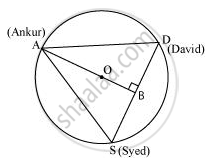# A circular park of radius 20 m is situated in a colony. Three boys Ankur, Syed and David are sitting at equal distance on its boundary each having a toy telephone in his hands to talk each other. Find the length of the string of each phone. - Mathematics

A circular park of radius 20 m is situated in a colony. Three boys Ankur, Syed and David are sitting at equal distance on its boundary each having a toy telephone in his hands to talk each other. Find the length of the string of each phone.

#### SolutionIt is given that AS = SD = DA

Therefore, ΔASD is an equilateral triangle.

Medians of equilateral triangle pass through the circum centre (O) of the equilateral triangle ASD. We also know that medians intersect each other in the ratio 2: 1. As AB is the median of equilateral triangle ASD, we can write

rArr(OA)/(OB) = 2/1

rArr(20m)/(OB) = 2/1

rArrOB=(20/2)m = 10m

∴ AB = OA + OB = (20 + 10) m = 30 m

In ΔABD,

AD^2= 900 + 1/4AD^2

3/4AD^2 = 900

AD^2 = 1200

AD = 20sqrt3

Therefore, the length of the string of each phone will be 20sqrt3" m"

Concept: Equal Chords and Their Distances from the Centre
Is there an error in this question or solution?

#### APPEARS IN

NCERT Class 9 Maths
Chapter 10 Circles
Exercise 10.4 | Q 6 | Page 179